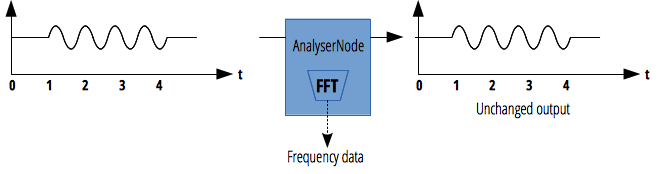# AnalyserNode

`AnalyserNode` 接口表示了一个可以提供实时频域和时域分析信息的节点。它是一个不对音频流作任何改动的 `AudioNode`，同时允许你获取和处理它生成的数据，从而创建音频可视化。

`AnalyzerNode` 只有一个输入和输出，即使未连接到输出它也能正常工作。## 构造函数

`AnalyserNode()`

## 属性

`AnalyserNode.fftSize`

`AnalyserNode.frequencyBinCount` 只读

`AnalyserNode.minDecibels` (en-US)

`AnalyserNode.maxDecibels` (en-US)

`AnalyserNode.smoothingTimeConstant`

## 方法

`AnalyserNode.getFloatFrequencyData()`

`AnalyserNode.getByteFrequencyData()`

`AnalyserNode.getFloatTimeDomainData()` (en-US)

`AnalyserNode.getByteTimeDomainData()`

## 例子

### 基础用法

js

``````var audioCtx = new (window.AudioContext || window.webkitAudioContext)();
var analyser = audioCtx.createAnalyser();

// ...

analyser.fftSize = 2048;
var bufferLength = analyser.frequencyBinCount;
var dataArray = new Uint8Array(bufferLength);
analyser.getByteTimeDomainData(dataArray);

// 获取 ID 为 "oscilloscope" 的画布
var canvas = document.getElementById("oscilloscope");
var canvasCtx = canvas.getContext("2d");

// 绘制一个当前音频源的示波器

function draw() {
drawVisual = requestAnimationFrame(draw);

analyser.getByteTimeDomainData(dataArray);

canvasCtx.fillStyle = "rgb(200, 200, 200)";
canvasCtx.fillRect(0, 0, canvas.width, canvas.height);

canvasCtx.lineWidth = 2;
canvasCtx.strokeStyle = "rgb(0, 0, 0)";

canvasCtx.beginPath();

var sliceWidth = (canvas.width * 1.0) / bufferLength;
var x = 0;

for (var i = 0; i < bufferLength; i++) {
var v = dataArray[i] / 128.0;
var y = (v * canvas.height) / 2;

if (i === 0) {
canvasCtx.moveTo(x, y);
} else {
canvasCtx.lineTo(x, y);
}

x += sliceWidth;
}

canvasCtx.lineTo(canvas.width, canvas.height / 2);
canvasCtx.stroke();
}

draw();
``````

Specification
Web Audio API
# AnalyserNode

## 浏览器兼容性

BCD tables only load in the browser